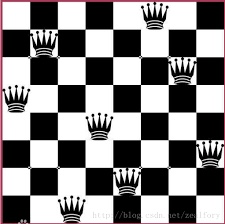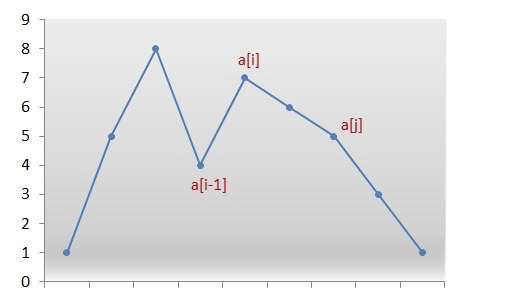# LeetCode 31：递归、回溯、八皇后、全排列一篇文章全讲清楚

2020/03/01 09:02

<br>

<br>

Next Permutation

## 难度

<font color=orange>Medium</font>

<br>

## 描述

<br>

Implement next permutation , which rearranges numbers into the lexicographically next greater permutation of numbers.

If such arrangement is not possible, it must rearrange it as the lowest possible order (ie, sorted in ascending order).

The replacement must be [in-place](http://en.wikipedia.org/wiki/In- place_algorithm) and use only constant extra memory.

Here are some examples. Inputs are in the left-hand column and its corresponding outputs are in the right-hand column.

<br>

### 样例

1,2,3 -> 1,3,2
3,2,1 -> 1,2,3
1,1,5 -> 1,5,1

<br>

## 介绍和分析

<br>

1 2 3
1 3 2
2 1 3
2 3 1
3 1 2
3 2 1


<br>

## 暴力

<br>

<br>

### 八皇后问题

<br>root
/
1
/
2
/
3
/
4
/
5
/ \
6   7
/ \
7   8
/     \
8       7


<br>

### 模板代码

<br>

def A():
do_something()
B()
do_something()


def A():
do_something()
A()
do_something()


def dfs():
for choice in all_choices():
record(choice)
dfs()
rollback(choice)


def dfs(depth):
if depth >= 8:
return
for choice in all_choices():
record(choice)
dfs(depth+1)
rollback(choice)


def dfs(n, queens, record):
# 记录答案与控制递归深度
if n >= 8:
# 由于Python当中传递的是引用，所以这里需要copy
# 否则当后面queens pop的时候，会影响record当中记录的值
record.append(queens.copy())
return

for i in range(8):
# 判断是否同列
if i in queens:
continue
# 判断是否同对角线
flag = False
for j in range(len(queens)):
if abs(n - j) == abs(i - queens[j]):
flag = True
break
if flag:
continue
# 递归与回溯
queens.append(i)
dfs(n+1, queens, record)
queens.pop()


def dfs(permutation, array, origin):
"""
origin记录原排列
mini  记录答案，即大于原排列的最小排列
"""
# 由于Python引用机制，在函数内部修改，外部不会生效
# 所以需要用全局变量来记录答案
global mini
# 当所有元素都已经放入permutation
if len(array) == 0:
# 判断是否合法，并且是最小的
if permutation > origin and permutation < mini:
# 因为后面permutation会执行pop
# 所以这里需要copy
mini = permutation.copy()
# 如果还有元素没有放入
for i in array.copy():
array.remove(i)
permutation.append(i)
dfs(permutation, array, origin)
array.append(i)
permutation.pop()


<br>

## 贪心

<br>class Solution:
def nextPermutation(self, nums: List[int]) -> None:
"""
Do not return anything, modify nums in-place instead.
"""
# 长度
n = len(nums)
# 记录图中i-1的位置
pos = n - 1
for i in range(n-1, 0, -1):
# 如果降序破坏，说明找到了i
if nums[i] > nums[i-1]:
pos = i-1
break

for i in range(n-1, pos, -1):
# 从最后开始找大于pos位置的
if nums[i] > nums[pos]:
# 先交换元素，在进行翻转
nums[i], nums[pos] = nums[pos], nums[i]
# 翻转[pos+1, n]区间
nums[pos+1:] = nums[n:pos:-1]
return

# 如果没有return，说明是最大的字典序，那么翻转整个数组
nums.reverse()0
0 收藏

### 作者的其它热门文章0 评论
0 收藏
0